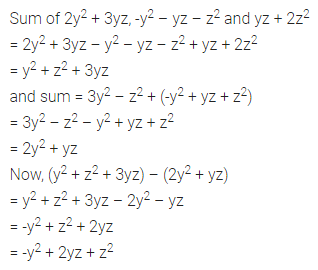# ML Aggarwal Class 7 Solutions for ICSE Maths Chapter 8 Algebraic Expressions Ex 8.2

## ML Aggarwal Class 7 Solutions for ICSE Maths Chapter 8 Algebraic Expressions Ex 8.2

Question 1.
(i) 7x, -3x
(ii) 6x, -11x
(iii) 5x2, -9x2
(iv) 3ab2, -5ab2
(v) $$\frac { 1 }{ 2 }$$ pq, $$\frac { -1 }{ 3 }$$ pq
(vi) 5x3y, $$\frac { -2 }{ 3 }$$ x3y
Solution:Question 2.
(i) 3x, -5x, 7x
(ii) 7xy, 2xy, -8xy
(iii) -2abc, 3abc, abc
(iv) 3mn, -5mn, 8mn, -4mn
(v) 2x3, 3x3, -4x3, -5x3
Solution: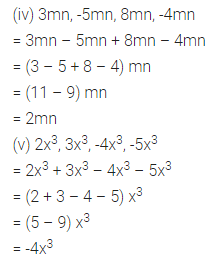Question 3.
Simplify the following combining like terms:
(i) 21b – 32 + 7b – 20b
(ii) 12m2 – 9m + 5m – 4m2 – 7m + 10
(iii) -z2 + 13z2 – 5z + 7z2 – 15z
(iv) 5x2y – 5x2 + 3yx2 – 3y2 + x2 – y2 + 8xy2 – 3y2
(v) p – (p – q) – (q – p) – q
(vi) 3a – 2b – ab – (a – b + ab) + 3ab + b – a
(vii) (3y2 + 5y – 4) – (8y – y2 – 4)
Solution:Question 4.
Find the sum of the following algebraic expressions:
(i) 5xy, -7xy, 3x2
(ii) 4x2y, -3xy2, -5xy2, 5x2y
(iii) -7mn + 5, 12mn + 2, 8mn – 8, -2mn – 3
(iv) a + b – 3, b – a + 3, a – b + 3
(v) 14x + 10y – 12xy – 13, 18 – 7x – 10y + 8xy, 4xy
(vi) 5m – 7n, 3n – 4m + 2, 2m – 3mn – 5
(vii) 3x3 – 5x2 + 2x + 1, 3x – 2x2 – x3, 2x2 – 7x + 9
(viii) 7a2 – 5a + 2, 3a2 – 7, 2a + 9, 1 + 2a – 5a2
Solution:Question 5.
Simplify the following:
(i) 2x2 + 3y2 – 5xy + 5x2 – y2 + 6xy – 3x2
(ii) 3xy2 – 5x2y + 7xy – 8xy2 – 4xy + 6x2y
(iii) 5x4 – 7x2 + 8x – 1 + 3x3 – 9x2 + 7 – 3x4 + 11x – 2 + 8x2
Solution:Question 6.
Subtract:
(i) -5y2 from y2
(ii) -7xy from -2xy
(iii) a(b – 5) from b(5 – a)
(iv) -m2 + 5mn from 4m2 – 3mn + 8
(v) 5a2 – 7ab + 5b2 from 3ab – 2b – 2b2
(vi) 4pq – 5q2 – 3p2 from 5p2 + 3q2 – pq
(vii) 7xy + 5x2 – 7y2 + 3 from 7x2 – 8xy + 3y2 – 5
(viii) 2x4 – 7x2 + 5x + 3 from x4 – 3x3 – 2x2 + 3
Solution: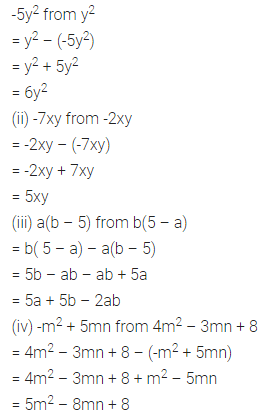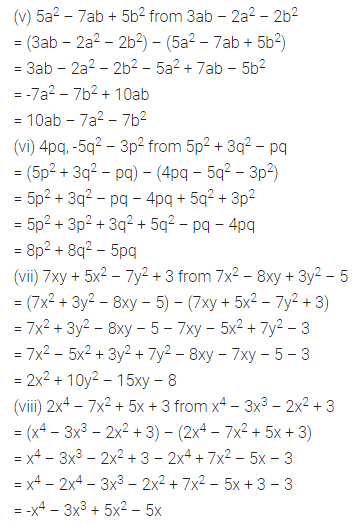Question 7.
Subtract p – 2q + r from the sum of 10p – r and 5p + 2q.
Solution:Question 8.
From the sum of 4 + 3x and 5 – 4x + 2x2, subtract the sum of 3x2 – 5x and -x2 + 2x + 5.
Solution:Question 9.
What should be added to x2 – y2 + 2xy to obtain x2 + y2 + 5xy?
Solution: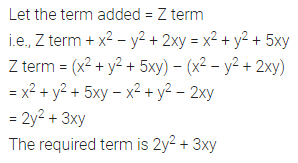Question 10.
What should be subtracted from -7mn + 2m2 + 3n2 to get m2 + 2mn + n2?
Solution: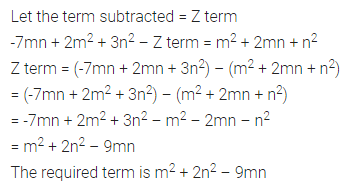Question 11.
How much is y4 – 12y2 + y + 14 greater than 17y3 + 34y2 – 51y + 68?
Solution: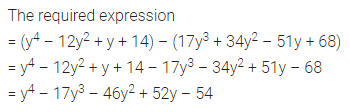Question 12.
How much does 93p2 – 55p + 4 exceed 13p3 – 5p2 + 17p – 90?
Solution:Question 13.
What should be taken away from 3x2 – 4y2 + 5xy + 20 to obtain -x2 – y2 + 6xy + 20?
Solution: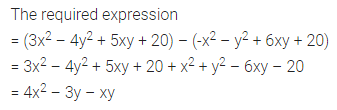Question 14.
From the sum of 2y2 + 3yz, -y2 – yz – z2 and yz + 2z2, subtract the sum of 3y2 – z2 and -y2 + yz + z2.
Solution: